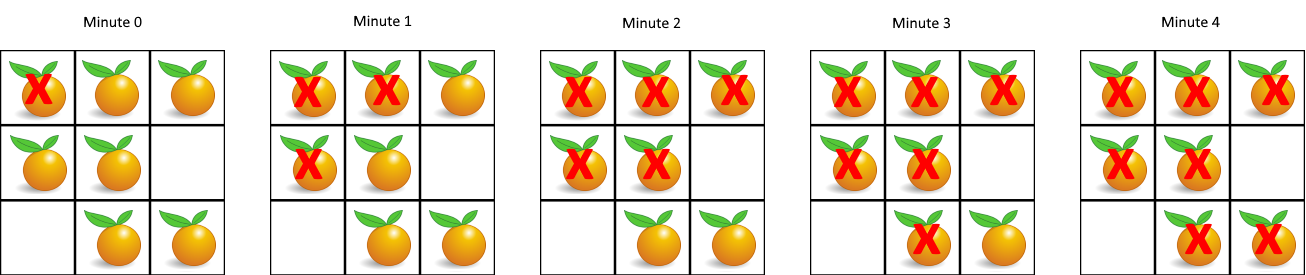Formatted question description: https://leetcode.ca/all/994.html

# 994. Rotting Oranges (Medium)

In a given grid, each cell can have one of three values:

• the value 0 representing an empty cell;
• the value 1 representing a fresh orange;
• the value 2 representing a rotten orange.

Every minute, any fresh orange that is adjacent (4-directionally) to a rotten orange becomes rotten.

Return the minimum number of minutes that must elapse until no cell has a fresh orange.  If this is impossible, return -1 instead.

Example 1:Input: [[2,1,1],[1,1,0],[0,1,1]]
Output: 4


Example 2:

Input: [[2,1,1],[0,1,1],[1,0,1]]
Output: -1
Explanation:  The orange in the bottom left corner (row 2, column 0) is never rotten, because rotting only happens 4-directionally.


Example 3:

Input: [[0,2]]
Output: 0
Explanation:  Since there are already no fresh oranges at minute 0, the answer is just 0.


Note:

1. 1 <= grid.length <= 10
2. 1 <= grid.length <= 10
3. grid[i][j] is only 0, 1, or 2.

Similar Questions:

## Solution 1. BFS

// OJ: https://leetcode.com/problems/rotting-oranges/

// Time: O(MN)
// Space: O(MN)
class Solution {
public:
int orangesRotting(vector<vector<int>>& A) {
int M = A.size(), N = A.size(), ans = 0, dirs = { {0,1},{0,-1},{1,0},{-1,0} };
queue<pair<int, int>> q;
for (int i = 0; i < M; ++i) {
for (int j = 0; j < N; ++j) {
if (A[i][j] == 2) q.emplace(i, j);
}
}
if (q.empty()) ans = 1;
while (q.size()) {
int cnt = q.size();
++ans;
while (cnt--) {
auto [x, y] = q.front();
q.pop();
for (auto &dir : dirs) {
int a = x + dir, b = y + dir;
if (a < 0 || a >= M || b < 0 || b >= N || A[a][b] != 1) continue;
A[a][b] = 2;
q.emplace(a, b);
}
}
}
for (auto &row : A) {
for (int x : row) {
if (x == 1) return -1;
}
}
return ans - 1;
}
};


Java

class Solution {
public int orangesRotting(int[][] grid) {
if (grid == null || grid.length == 0 || grid.length == 0)
return 0;
int rows = grid.length, columns = grid.length;
final int BLOCK = -1;
final int WHITE = 0;
final int GRAY = 1;
final int BLACK = 2;
int[][] colors = new int[rows][columns];
int[][] distances = new int[rows][columns];
for (int i = 0; i < rows; i++) {
for (int j = 0; j < columns; j++) {
int value = grid[i][j];
if (value == 2) {
distances[i][j] = 0;
colors[i][j] = GRAY;
queue.offer(new int[]{i, j});
} else if (value == 1) {
distances[i][j] = Integer.MAX_VALUE;
colors[i][j] = WHITE;
} else {
distances[i][j] = -1;
colors[i][j] = BLOCK;
}
}
}
int[][] directions = { {-1, 0}, {1, 0}, {0, -1}, {0, 1} };
while (!queue.isEmpty()) {
int[] cell = queue.poll();
int row = cell, column = cell;
int distance = distances[row][column];
for (int[] direction : directions) {
int newRow = row + direction, newColumn = column + direction;
if (newRow >= 0 && newRow < rows && newColumn >= 0 && newColumn < columns) {
if (colors[newRow][newColumn] == WHITE) {
distances[newRow][newColumn] = distance + 1;
colors[newRow][newColumn] = GRAY;
queue.offer(new int[]{newRow, newColumn});
}
}
}
colors[row][column] = BLACK;
}
int time = 0;
for (int i = 0; i < rows; i++) {
for (int j = 0; j < columns; j++) {
time = Math.max(time, distances[i][j]);
if (time == Integer.MAX_VALUE)
return -1;
}
}
return time == Integer.MAX_VALUE ? -1 : time;
}
}